x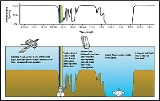Opacity (optics)Encyclopedia
Opacity is the measure of impenetrability to electromagnetic
Electromagnetic radiation is a form of energy that exhibits wave-like behavior as it travels through space...

or other kinds of radiation
In physics, radiation is a process in which energetic particles or energetic waves travel through a medium or space. There are two distinct types of radiation; ionizing and non-ionizing...

, especially visible light
Light
Light or visible light is electromagnetic radiation that is visible to the human eye, and is responsible for the sense of sight. Visible light has wavelength in a range from about 380 nanometres to about 740 nm, with a frequency range of about 405 THz to 790 THz...

. In radiative transfer
Radiative transfer is the physical phenomenon of energy transfer in the form of electromagnetic radiation. The propagation of radiation through a medium is affected by absorption, emission and scattering processes. The equation of radiative transfer describes these interactions mathematically...

, it describes the absorption and scattering of radiation in a medium
Transmission medium
A transmission medium is a material substance that can propagate energy waves...

, such as a plasma
Plasma (physics)
In physics and chemistry, plasma is a state of matter similar to gas in which a certain portion of the particles are ionized. Heating a gas may ionize its molecules or atoms , thus turning it into a plasma, which contains charged particles: positive ions and negative electrons or ions...

, dielectric
Dielectric
A dielectric is an electrical insulator that can be polarized by an applied electric field. When a dielectric is placed in an electric field, electric charges do not flow through the material, as in a conductor, but only slightly shift from their average equilibrium positions causing dielectric...

, shielding material, glass, etc. An opaque object is neither transparent (allowing all light to pass through) nor translucent (allowing some light to pass through). When light strikes an interface between two substances, in general some may be reflected, some absorbed, some scattered, and the rest transmitted (also see refraction
Refraction
Refraction is the change in direction of a wave due to a change in its speed. It is essentially a surface phenomenon . The phenomenon is mainly in governance to the law of conservation of energy. The proper explanation would be that due to change of medium, the phase velocity of the wave is changed...

). Reflection can be diffuse
Diffuse reflection
Diffuse reflection is the reflection of light from a surface such that an incident ray is reflected at many angles rather than at just one angle as in the case of specular reflection...

, for example light reflecting off a white wall, or specular
Specular reflection
Specular reflection is the mirror-like reflection of light from a surface, in which light from a single incoming direction is reflected into a single outgoing direction...

, for example light reflecting off a mirror. An opaque substance transmits no light, and therefore reflects, scatters, or absorbs all of it. Both mirror
Mirror
A mirror is an object that reflects light or sound in a way that preserves much of its original quality prior to its contact with the mirror. Some mirrors also filter out some wavelengths, while preserving other wavelengths in the reflection...

s and carbon black
Carbon black
Carbon black is a material produced by the incomplete combustion of heavy petroleum products such as FCC tar, coal tar, ethylene cracking tar, and a small amount from vegetable oil. Carbon black is a form of amorphous carbon that has a high surface-area-to-volume ratio, although its...

are opaque. Opacity depends on the frequency
Frequency
Frequency is the number of occurrences of a repeating event per unit time. It is also referred to as temporal frequency.The period is the duration of one cycle in a repeating event, so the period is the reciprocal of the frequency...

of the light being considered. For instance, some kinds of glass
Glass
Glass is an amorphous solid material. Glasses are typically brittle and optically transparent.The most familiar type of glass, used for centuries in windows and drinking vessels, is soda-lime glass, composed of about 75% silica plus Na2O, CaO, and several minor additives...

, while transparent in the visual range, are largely opaque to ultraviolet
Ultraviolet
Ultraviolet light is electromagnetic radiation with a wavelength shorter than that of visible light, but longer than X-rays, in the range 10 nm to 400 nm, and energies from 3 eV to 124 eV...

light. More extreme frequency-dependence is visible in the absorption lines of cold gas
Gas
Gas is one of the three classical states of matter . Near absolute zero, a substance exists as a solid. As heat is added to this substance it melts into a liquid at its melting point , boils into a gas at its boiling point, and if heated high enough would enter a plasma state in which the electrons...

es. Opacity can be quantified in many ways; for example, see the article mathematical descriptions of opacity
Mathematical descriptions of opacity
When an electromagnetic wave travels through a medium in which it gets absorbed , it undergoes exponential decay as described by the Beer–Lambert law. However, there are many possible ways to characterize the wave and how quickly it is absorbed...

.

For general information on what makes an object or medium opaque, see the articles on absorption
In physics, absorption of electromagnetic radiation is the way by which the energy of a photon is taken up by matter, typically the electrons of an atom. Thus, the electromagnetic energy is transformed to other forms of energy for example, to heat. The absorption of light during wave propagation is...

, reflection
Reflection (physics)
Reflection is the change in direction of a wavefront at an interface between two differentmedia so that the wavefront returns into the medium from which it originated. Common examples include the reflection of light, sound and water waves...

, and scattering
Light scattering
Light scattering is a form of scattering in which light is the form of propagating energy which is scattered. Light scattering can be thought of as the deflection of a ray from a straight path, for example by irregularities in the propagation medium, particles, or in the interface between two media...

. These are the processes that lead to opacity.

## Quantitative definition

The words "opacity" and "opaque" are often used as colloquial terms for objects or media with the properties described above. However, there is also a specific, quantitative definition of "opacity", used in astronomy, plasma physics, and other fields, given here.

In this use, "opacity" is another term for the mass attenuation coefficient
Mass attenuation coefficient
The mass attenuation coefficient is a measurement of how strongly a chemical species or substance absorbs or scatters light at a given wavelength, per unit mass...

(or, depending on context, mass absorption coefficient, the difference is described here)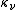at a particular frequencyof electromagnetic radiation.

More specifically, if a beam of light with frequencytravels through a medium with opacityand mass density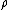, both constant, then the intensity will be reduced with distance x according to the formula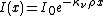where
• x is the distance the light has traveled through the medium
•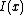is the intensity of light remaining at distance x
•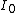is the initial intensity of light, at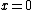For a given medium at a given frequency, the opacity has a numerical value that may range between 0 and infinity, with units of length2/mass.

### Planck and Rosseland opacity

It is customary to define the average opacity, calculated using a certain weighting scheme. Planck opacity uses normalized Planck black body radiation energy density distribution
Planck's law of black body radiation
In physics, Planck's law describes the amount of energy emitted by a black body in radiation of a certain wavelength . The law is named after Max Planck, who originally proposed it in 1900. The law was the first to accurately describe black body radiation, and resolved the ultraviolet catastrophe...

as the weighting function, and averagesdirectly. Rosseland opacity (after Svein Rosseland
Svein Rosseland
Svein Rosseland was a Norwegian astrophysicist and a pioneer in the field of theoretical astrophysics. -Biography:...

), on the other hand, uses a temperature derivative of Planck distribution
Planck's law of black body radiation
In physics, Planck's law describes the amount of energy emitted by a black body in radiation of a certain wavelength . The law is named after Max Planck, who originally proposed it in 1900. The law was the first to accurately describe black body radiation, and resolved the ultraviolet catastrophe...

(normalized) as the weighting function, and averages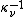,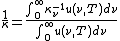.
The photon mean free path is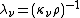. The Rosseland opacity is derived in the diffusion approximation to the radiative transport equation. It is valid whenever the radiation field is isotropic over distances comparable to or less than a radiation mean free path, such as in local thermal equilibrium.
In practice, the mean opacity for Thomson electron scattering
Thomson scattering
Thomson scattering is the elastic scattering of electromagnetic radiation by a free charged particle, as described by classical electromagnetism. It is just the low-energy limit of Compton scattering: the particle kinetic energy and photon frequency are the same before and after the scattering...

is: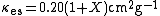where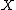is the hydrogen mass fraction.
For nonrelativistic thermal bremsstrahlung
Bremsstrahlung
Bremsstrahlung is electromagnetic radiation produced by the deceleration of a charged particle when deflected by another charged particle, typically an electron by an atomic nucleus. The moving particle loses kinetic energy, which is converted into a photon because energy is conserved. The term is...

, or free-free transitions, it is: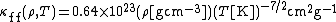.
The Rosseland mean absorption coefficient including both scattering and absorption (also called the extinction coefficient) is: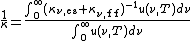.

• Absorption (electromagnetic radiation)
In physics, absorption of electromagnetic radiation is the way by which the energy of a photon is taken up by matter, typically the electrons of an atom. Thus, the electromagnetic energy is transformed to other forms of energy for example, to heat. The absorption of light during wave propagation is...

• Mathematical descriptions of opacity
Mathematical descriptions of opacity
When an electromagnetic wave travels through a medium in which it gets absorbed , it undergoes exponential decay as described by the Beer–Lambert law. However, there are many possible ways to characterize the wave and how quickly it is absorbed...

• Molar absorptivity
Molar absorptivity
The molar absorption coefficient, molar extinction coefficient, or molar absorptivity, is a measurement of how strongly a chemical species absorbs light at a given wavelength...

• Reflection (physics)
Reflection (physics)
Reflection is the change in direction of a wavefront at an interface between two differentmedia so that the wavefront returns into the medium from which it originated. Common examples include the reflection of light, sound and water waves...

• Scattering theory
Scattering theory
In mathematics and physics, scattering theory is a framework for studying and understanding the scattering of waves and particles. Prosaically, wave scattering corresponds to the collision and scattering of a wave with some material object, for instance sunlight scattered by rain drops to form a...